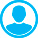NEW PROBLEMS OF DESCRIPTIVE GEOMETRYAbstract (English):
Complex geometry consists of Euclidean E-geometry (circle geometry) and pseudo-Euclidean M-geometry (hyperbola geometry). Each of them individually determines an open system in which a correctly posed problem may give no solution. Analytical geometry is an example of a closed system, in which the previously mentioned problem always gives a solution as a complex number, whose one of the parts may turn out to be zero. Development of imaginary solutions and imaginary figures is a new task for descriptive geometry. Degenerated conics and quadrics set up a new class of figures and a new class of descriptive geometry’s problems. For example, a null circle, null sphere, null cylinder, and a cone as a hyperboloid degenerated to an asymptote. The last ones necessarily lead to imaginary solutions in geometric operations. In this paper it has been shown that theorems formulated in one geometry are also valid in conjugate geometry as well, while the same figures of conjugated geometries visually look different. So imaginary points exist only by pairs, the imaginary circle is not round one, the centers of dissimilar circles’ similarity do not belong to the centerline and other examples. For solution, a number of problems on geometric relations, and operations with degenerated conics and quadrics, as well as several problems from 4D-geometry are proposed. Solutions for above mentioned problems are given in section 9. In this paper some examples of new problems for descriptive geometry have been considered. It has been shown that the new problems require access to a complex space. New figures consist of two parts, a real figure and a figure of its imaginary complement.

Keywords:
analytical figure, synthetic figure, imaginary sphere, imaginary cylinder, degenerated surfaces, null sphere, null cylinder, imaginary complement, isotropes, contact, intersection, 4D figures

1. Voloshinov D.V. Vizual`no-graficheskoe proektirovanie edinoj konstruktivnoj modeli dlya resheniya analogov zadachi Apolloniya s uchetom mnimy`x geometricheskix obrazov [Visual and graphic design of a single structural model for solving the analogs of the Apollonius problem taking into account imaginary geometric images]. Geometriya i grafika [Geometry and Graphics]. 2018, V. 6, I. 2, pp. 23–46. – DOI: 10.12737/article_5b559c70becf44.21848537. (in Russian)

2. Voloshinov D.V. Ediny`j konstruktivny`j algoritm postroeniya fokusov krivy`x vtorogo poryadka [Unified constructive algorithm for constructing foci of second-order curves]. Geometriya i grafika [Geometry and Graphics]. 2018, V. 6, I. 2, pp. 47–54. – DOI: 10.12737/article_5b559dc3551f95.26045830. (in Russian)

3. Girsh A.G. Kompleksnaya geometriya — evklidova i psevdoevklidova [Complex geometry — Euclidean and pseudo-Euclidean]. Moscow, IPTs ≪Maska≫ Publ., 2013. 216 p. (in Russian)

4. Girsh A.G. Mnimosti v geometrii [Imaginaries in Geometry]. Geometriya i grafika [Geometry and Graphics]. 2014, V. 2, I. 2, pp. 3–8. DOI: 10.12737/5583. (in Russian)

5. Girsh A.G. Naglyadnaya mnimaya geometriya [Transparent imaginary geometry]. Moscow, IPTs ≪Maska≫ Publ., 2008. 216 p. (in Russian)

6. Girsh A.G. Nachala kompleksnoj geometrii. Sbornik zadach po kompleksnoj geo-metrii s resheniyami [The Beginning of complex geometry. Collection of problems on complex geometry with solutions]. Kassel`, 2012. 191 p. (in Russian)

7. Girsh A.G. Nachala kompleksnoj geometrii. Izbranny`e zadachi kompleksnoj geometrii s resheniyami. Chast` II – 3D [The Beginning of complex geometry. Selected problems of complex geometry with solutions. Part II-3D]. Kassel`, 2014. 112 p. (in Russian)

8. Girsh A.G. Poverxnost` ot vrashheniya okruzhnosti [the Surface from the rotation of the circle] Geometriya i grafika. [Geometry and Graphics]. 2017, V. 5, I. 1, pp. 32-35. DOI: 10.12737/25121. (in Russian)

9. Girsh A.G., Korotkij V.A. Graficheskie algoritmy` rekonstrukcii krivoj vto-rogo poryadka, zadannoj mnimy`mi e`lementami [Graphic algorithms for reconstruction of a second-order curve given by imaginary elements]. Geometriya i grafika. [Geometry and Graphics]. 2016, V. 4, I. 4, pp. 19–30. DOI: 10.12737/22840. (in Russian)

10. Girsh A.G., Korotkij V.A. Mnimy`e tochki v dekartovoj sisteme koordinat [Imaginary points in the Cartesian coordinate system]. Geometriya i grafika. [Geometry and Graphics]. 2019, V. 7, I. 3, pp. 28-35. DOI: 10.12737/article_5dce651d80b827.498308216. (in Russian)

11. Ivanov G.S. O zadachakh nachertatel'noy geometrii s mnimymi resheniyami [On the tasks of descriptive geometry with imaginary solutions]. Geometriya i grafika [Geometry and Graphics]. 2015, V. 3, I. 2, pp. 3–8. DOI: 10.12737/12163. (in Russian)

12. Kleyn F. Vysshaya geometriya [Higher geometry]. Moscow, URSS Publ., 2004. 400 p. (in Russian)

13. Kleyn F. Elementarnaya matematika s tochki zreniya vysshey [Elementary mathematics from the point of view of the highest]. Moscow, Nauka Publ., 1987, V. 2, 416 p. (in Russian)

14. Kuzneczov N.S. Nachertatel`naya geometriya [Descriptive geometry]. Moscow, Vy`sshaya shkola Publ., 1981. 262 p. (in Russian)

15. Lyashkov A.A. Panchuk K.L., Varepo L.G. Osobennost` otobrazheniya giperpoverxnosti chety`rexmernogo prostranstva [Feature of hypersurface mapping of a four-dimensional space]. Geometriya i grafika [Geometry and Graphics]. 2017, V. 5, I. 3, pp. 3–10. DOI: 10.12737/article_59bfa3078af4c1.45321238. (in Russian)

16. Peklich V.A. Vysshaya nachertatel'naya geometriya [Higher descriptive geometry]. Moscow, ASV Publ., 2000. 344 p. (in Russian)

17. Peklich V.A. Mnimaya nachertatel'naya geometriya [Imaginary descriptive geometry]. Moscow, ASV Publ., 2007. 104 p. (in Russian)

18. Savel'ev Yu.A. Grafika mnimykh chisel [Graphic imaginary numbers]. Geometriya i grafika [Geometry and Graphics]. 2013, V. 1, I. 1, pp. 22–23. DOI: 10.12737/465. (in Russian)

19. Florenskij P.A. Mnimosti v geometrii [Mnimosti in geometry]. Moscow: Lazur` Publ., 1991. 96 p. (in Russian)

20. Reye Th. Geometrie der Lage. 1. Abteilung. – Leipzig, 1882, 215 S.